Courses

# Test Level 1: Chemical Bonding & Molecular Structure

## 25 Questions MCQ Test Chemistry Class 11 | Test Level 1: Chemical Bonding & Molecular Structure

Description
This mock test of Test Level 1: Chemical Bonding & Molecular Structure for JEE helps you for every JEE entrance exam. This contains 25 Multiple Choice Questions for JEE Test Level 1: Chemical Bonding & Molecular Structure (mcq) to study with solutions a complete question bank. The solved questions answers in this Test Level 1: Chemical Bonding & Molecular Structure quiz give you a good mix of easy questions and tough questions. JEE students definitely take this Test Level 1: Chemical Bonding & Molecular Structure exercise for a better result in the exam. You can find other Test Level 1: Chemical Bonding & Molecular Structure extra questions, long questions & short questions for JEE on EduRev as well by searching above.
QUESTION: 1

### The formation of the Cl2 molecule can be understood in terms of the sharing of a pair of electrons between the two chlorine atoms, each chlorine atom contributing one electron to the shared pair. Choose the most appropriate name of the bond that is formed:

Solution:
• Cl2 is formed by single covalent bond. Two chlorine atoms will each share one electron to get a full outer shell and form a stable Cl2 molecule.
• By sharing the two electrons where the shells touch each chlorine atom can count 8 electrons in its outer shell. These full outer shells with their shared electrons are now stable and the Cl2 molecule will not react further with other chlorine atoms. One pair of shared electrons form a single covalent bond.
QUESTION: 2

### In the case of a heteronuclear molecule like HF, the shared electron pair between the two atoms gets displaced more towards fluorine since the electronegativity of fluorine is far greater than that of hydrogen. This results in the molecule a:

Solution:

Because of slight shifting of electron towards more electronegative element like F in HF brings a small amount of negative charge on F and positive charge on H which results in dipole moment.

QUESTION: 3

### Among the following the maximum covalent character is shown by the compound:

Solution:
• As the cation charge increases, the covalent character also increases.
• According to Fajans rule, a cation with a greater charge and smaller size favours covalency.
QUESTION: 4

Which of the following angle corresponds to sp2  hybridisation?

Solution:

sp2 hybridisation gives three sp2 hybrid orbitals which are planar triangular forming an angle of 120° with each other.

QUESTION: 5

Which of the following statements are not correct?

Solution:

NaCl is not good conductor in solid state. It conducts electricity only in molten state.

QUESTION: 6

When a gas phase atom in its ground state gains an electron. This is called:

Solution:

Electron gain enthalpy is the energy released when an electron is gained by an isolated gaseous atom to form an isolated gaseous anion.

QUESTION: 7

Elements in which apart from 3s and 3p orbitals, 3d orbitals also available for bonding In a number of compounds of these elements there are more than eight valence electrons around the central atom. One such example is:

Solution:

In H2SO4, S has more than 8 electrons in the valence shell.

QUESTION: 8

The number of types of bonds between two carbon atoms in calcium carbide is:

Solution:

CaC2 can be divided into two ions: Ca2+, C22-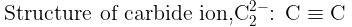• There is a triple bond, between two carbon.
• Among three bonds one is sigma, bond and the remaining two are pi bond.
QUESTION: 9

The electronic configuration of the outer most shell of the most electronegative element is:

Solution:
• The most electronegative element is F. Its atomic number is 9.
• So, the electronic configuration is 2s22p5.
QUESTION: 10

Rank the bonds in the set C=O, C-O, C≡O, in the order of decreasing bond length:

Solution:

C≡O has triple bond so has minimum bond length.

QUESTION: 11

A qualitative measure of the stability of an ionic compound is provided:

Solution:

The stability of an ionic bond is directly proportional to lattice energy.

QUESTION: 12

The shift in electron density is symbolized by the crossed arrow in the below diagram. It depicts: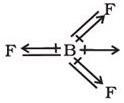Solution:

Arrows represent the direction of the dipole moment.

QUESTION: 13

Which one of the following is paramagnetic?

Solution:

The Lewis structure of NO molecule can be represented as: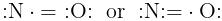• It is observed that the total no. of unbonded electrons is odd.
• Therefore, there must be an incompletely filled subshell.

Thus, NO is paramagnetic in nature.

QUESTION: 14

Using MO theory predicts which of the following species has the shortest bond length?

Solution:

Bond length is inversely proportional to bond order.

O2+2 has bond highest bond order as electrons are removed from antibonding MO.

QUESTION: 15

Rank the bonds in the set C=O, C-O, C≡O in order of decreasing bond strength:

Solution:

Bond order is directly proportional to bond strength. The higher the bond order, the stronger the pull between the two atoms and the shorter the bond length.

Correct order is: C≡O > C=O > C-O

QUESTION: 16

Bond lengths are lower in elements having:

Solution:

Single bond has higher bond length than multiple bond.

QUESTION: 17

In BF3, the molecule below the dipole moment is zero although the B-F bonds are oriented at an angle of 1200 to one another. Net dipole moment in BF3 molecule is:

Solution:

In the BF3 molecule, the dipole moment is zero because the B-F bonds are oriented at an angle of 1200 to one another resulting, all dipoles cancel out each other.

QUESTION: 18

Hybridization of Cand Cof H3C − CH = C = CH − CHare:

Solution: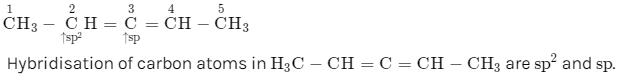C2 has 1 double bond, so it is sp2 hybridized while C3 has 2 double bonds so it is sp hybridized.

QUESTION: 19

Which one of the following pairs of species has the same bond order?

Solution:
• The species which have the same number of total electrons will have the same bond order.
• CNand NO+ each have 14 electrons and they will have the same bond order.
QUESTION: 20

Quartz is very hard and melts at 1550C . Reason is:

Solution:

Quartz/silica have extended covalent bond network.

QUESTION: 21

H-O-H bond angle in water is:

Solution:

Due to the presence of two lone pairs on O in H2O bond angle reduce to 104º from 109º.

QUESTION: 22

The shape of the below molecule is: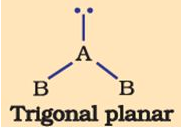Solution:

The order of Repulsion: lone pair-lone pair > lone pair-bond pair > bond pair-bond pair

Due to the extra lone pair electron, the shape becomes bent.

QUESTION: 23

The sp3d2 hybridization of central atom of a molecule would lead to

Solution:

It will lead to an octahedral structure assuming that there are no unpaired electrons in the central atom. In case there are any , you have to consider the shape that bonding pairs of electrons are in.

so sp3d2 hybridization will lead to octahedral geometry.

QUESTION: 24

CO is isoelectronic with

Solution:

CO , N2 and NO+ have same number of electrons = 14.

QUESTION: 25

Rank the following bonds in order of increasing polarity: H-N, H-O, H-C.

Solution:

O is more electronegative than N which is more electronegative than C.